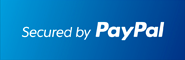** New: ECONOMICS , DIFFERENTIAL EQUATIONS **         ** SPECIALS for bulk apps **         ** Bestsellers: CALCULUS , SAT , PHYSICS , CHEM , STOCKS & PORTFOLIOS , ALGEBRA **# #1 Algebra Solver for the TI-89 Calculator

## Solve Algebra problems Step by Step.

### Works With TI92 Plus TI89 TI89 Titanium Voyage 200At the end of the PayPal checkout, you will be sent an email containing your key and software.Description
• The most comprehensive Algebra APP for calculators.
• Covers the ENTIRE Algebra curriculum: Algebra, Functions and their Analysis, Trig, Geometry, Complex Numbers, Matrices, Exponential/Logarithmic Functions, Probabilities, Combinatorics, Sequences, Induction, Sigma Notation, etc.
• Users have boosted their Algebra knowledge & grades.
• View Rules, Theorems, Identities.
• Ideal for quick review in all Algebra classes as well as classes such asTrigonometry, Calculus, Physics, Chemistry, Biology, Discrete Mathematics, Geometry, Complex Numbers.
• Easy to use. Just plug in the equation or numbers and the correct answer shows.
• Step by Step - Complete the Square
• Step by Step - Partial Fractions
• Step by Step - Complex Numbers
• Step by Step - Powers
• Step by Step - Roots
• Step by Step - Synthetic Division
• Step by Step - Radicals
• Logarithm and Exponential Solver
• Rewrite Logarithms into their Exponential Form and back: log_b(y)=x <--> y=b^x
• Expand and Condense Logarithms Step by Step
• Rule of 72, Change of Logarithm base
• Effective Interest rates
• Euler Number as Limit Definition.
• Money Growth Solver
• and much more that is needed for Algebra..

Visit the Classic Site at www.ti89.com/index.htm for more details.

Documentation